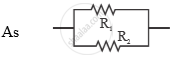Advertisement Remove all ads

# Two Tungsten Bulbs of Power 50 W and 60 W Work on 220 V Potential Difference. If They Are Connected in Parallel, How Much Current Will Flow in the Main Conductor? - Science and Technology 1

Answer in Brief

Two tungsten bulbs of power 50 W and 60 W work on 220 V potential difference. If they are connected in parallel, how much current will flow in the main conductor?

Advertisement Remove all ads

#### Solution

P1 = 50W

"R"_1 = "V"^2/"P"_1 = (220)^2/50

4840/5 Omega

P2 =60 W

"R"_2 = "V"_2/"P"_2 = (220)^2/60 4840/6 Omega1/"R"_"P" = 1/"R"_1 +1/"R"_2 = 5/4840+6/4840 = 11/4840 = 1/440

"R"_"P" = 440  Omega

"I" ="V"/"R"_"p" = 220/440  "A"=  0.5"A"

Concept: Energy Transfer in an Electric Circuit
Is there an error in this question or solution?
Advertisement Remove all ads

#### APPEARS IN

Advertisement Remove all ads
Advertisement Remove all ads
Share
Notifications

View all notifications

Forgot password?